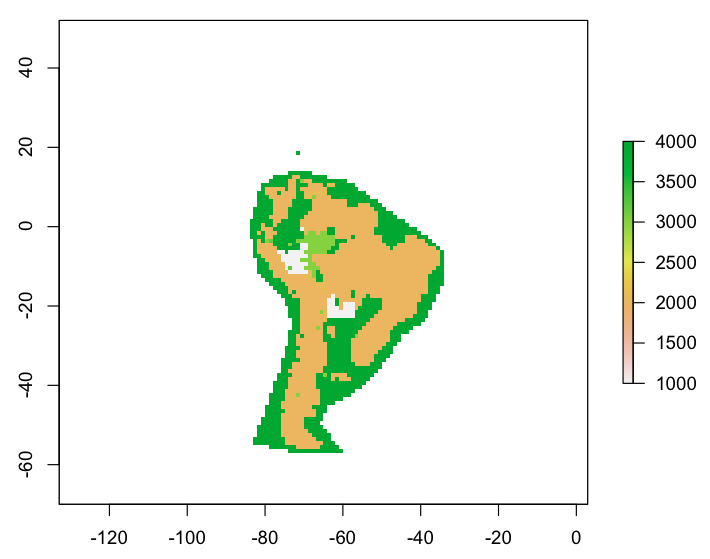# Using R to Create a Winner-Takes-All Raster from Point DataI am to the point in my dissertation where I need to summarize a fair amount of data.  The full data structure will be in the final manuscript, but for now suffice it to say that I am storing data as latitude/longitude pairs in a flat-file database (and using Panorama Sheets to query and modify it as needed).  Point data can be exported from this database as a CSV (actually tab-separated) file and converted to a shapefile in QGIS.

For this project, the globe is divided into 1×1 degree cells.  Each cell in my study area contains at least one vector point in my locality shapefile (exported from te database, above).  Many cells contain multiple points, each of which may represent a locality interpreted as representing a different paleoenvironment than the others.  I am using R to summarize these data points into a raster for each time slice I wish to study.

The method below emplys a winner-takes-all method, which is to say that during conversion from point data to raster, the points within each cell are evaluated for the values in the field ‘env_id’ (environment ID number, since we can’t use text in a raster), and the most frequent value of ‘env_id’ “wins” the cell and becomes the value burned into the output raster.

```### Converting a point shapefile (with environment in numeric
### field env_id) into a raster
### Winner-takes-all.

# Load libraries for raster, maptools and mode
library(raster);
library(maptools);
library(modeest);

# Read in the locality point shapefile

# Make the base raster.
basegrid<-raster(ncols=360, nrows=180);

# Create a subset for the age you are looking at
locs_subset<-subset(locs,locs\$time_slice==10);

# Convert the locality shapefile to raster.  Use the most frequent
# value of numeric field 'env_id'.
r<-rasterize(locs_subset, basegrid, field='env_id', fun=function(x, ...){mfv(x)});

# View the plot (centered on SA)
plot(r,xlim=c(-100,-30),ylim=c(-70,52));

# View the plot (all)
# plot(r);

# Write the raster to file
writeRaster(r,"10 Ma.gtiff","GTiff");
```

Output from R looks something like the top image, and can be manipulated as any other raster in QGIS etc.  Scale bar shows the numeric values used to represent different paleoenvironments in the raster; it’s not actually a continuous scale but I didn’t have a need to change the default R output.